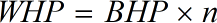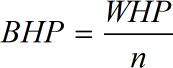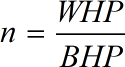Request a Tool

Pump Efficiency Calculator

The ratio of energy transformed into meaningful work to the energy applied to the pump shaft or the energy differential in the water is referred to as pump efficiency.

Water Horsepower
0

Formula• WHP = Water Horsepowe
• n = Pump Efficiency
• BHP = Brake Horsepower

Defination / Uses

The water horsepower is the amount of power necessary to drive a centrifugal pump. It can be calculated by its flow rate and total head.

Brake Horsepower
0

Formula• BHP = Brake Horsepower
• WHP = Water Horsepowe
• n = Pump Efficiency

Defination / Uses

The entire amount of horsepower or energy necessary to overcome the breaking force in a centrifugal pump is referred to as brake horsepower.

Pump Efficiency
0

Formula• n = Pump Efficiency
• BHP = Brake Horsepower
• WHP = Water Horsepowe

Defination / Uses

Pump efficiency is termed as the ratio of the power or energy imparted on the fluid by the pump in relation to the power supplied to drive the pump.

How to use pump efficiency calcultor?

There are only two basic steps.

• First select the option from dropdown. What you need to calculate.
• Then add the other values in their corresponding fields.